Chemistry: CUET Mock Test - 1

# Chemistry: CUET Mock Test - 1

Test Description

## 40 Questions MCQ Test CUET Science Subjects Mock Tests | Chemistry: CUET Mock Test - 1

Chemistry: CUET Mock Test - 1 for Class 12 2023 is part of CUET Science Subjects Mock Tests preparation. The Chemistry: CUET Mock Test - 1 questions and answers have been prepared according to the Class 12 exam syllabus.The Chemistry: CUET Mock Test - 1 MCQs are made for Class 12 2023 Exam. Find important definitions, questions, notes, meanings, examples, exercises, MCQs and online tests for Chemistry: CUET Mock Test - 1 below.
Solutions of Chemistry: CUET Mock Test - 1 questions in English are available as part of our CUET Science Subjects Mock Tests for Class 12 & Chemistry: CUET Mock Test - 1 solutions in Hindi for CUET Science Subjects Mock Tests course. Download more important topics, notes, lectures and mock test series for Class 12 Exam by signing up for free. Attempt Chemistry: CUET Mock Test - 1 | 40 questions in 45 minutes | Mock test for Class 12 preparation | Free important questions MCQ to study CUET Science Subjects Mock Tests for Class 12 Exam | Download free PDF with solutions
 1 Crore+ students have signed up on EduRev. Have you?
Chemistry: CUET Mock Test - 1 - Question 1

### Which of the following is regarded as the ‘repeatable entity’ of a 3D crystal structure?

Detailed Solution for Chemistry: CUET Mock Test - 1 - Question 1

Unit cell is the smallest entity of a crystal lattice which, when repeated in space (3 dimensions) generates the entire crystal lattice. Lattice comprises of the unit cells which hold all the particles in a particular arrangement in 3 dimensions. Crystal is a piece of homogenous solid and Bravais indices are used to define planes in crystal lattices in the hexagonal system.

Chemistry: CUET Mock Test - 1 - Question 2

### In primitive unit cubic cell, only _______ of an atom (or ion or molecule) belongs to a particular unit cell.

Detailed Solution for Chemistry: CUET Mock Test - 1 - Question 2

Explanation: In primitive unit cubic cell, each atom at the corner is shared between 8 adjacent unit cells. Thus, only 1/8th of an atom (or ion or molecule) belongs to a particular unit cell.

Chemistry: CUET Mock Test - 1 - Question 3

### What kind of order is present in a solid for it to be a perfectly crystalline solid?

Detailed Solution for Chemistry: CUET Mock Test - 1 - Question 3

Only short range order is a feature of amorphous solids. Crystalline solids exhibit both short and long range order. Crystalline solids are formed by repetitive arrangement of unit cells.

Chemistry: CUET Mock Test - 1 - Question 4

Which of the following statements regarding Ideal solutions is false?

Detailed Solution for Chemistry: CUET Mock Test - 1 - Question 4

An ideal solution is the solution in which each component obeys Raoult’s law under all conditions of temperature and concentrations. An ideal solution will satisfy the following conditions:
I. There will be no change in volume on mixing the components, i.e., ΔVmixing = 0.
II. There will be no change in enthalpy (i.e., no heat is evolved or absorbed) when the two components are mixed, i.e., ΔHmixing = 0.

Chemistry: CUET Mock Test - 1 - Question 5

Which of the following unit cells has constituent particles occupying the corner positions only?

Detailed Solution for Chemistry: CUET Mock Test - 1 - Question 5

According to classification of unit cells, a primitive unit cell is one which has all constituent particles located at its corners. BCC has one particle present at the center including the corners. FCC has an individual cell shared between the faces of adjacent cells. End centered cells have cells present at centers of two opposite faces.

Chemistry: CUET Mock Test - 1 - Question 6

The total number of atoms in one unit cell of primitive unit cubic cell is ______ atom(s).

Detailed Solution for Chemistry: CUET Mock Test - 1 - Question 6

In primitive cubic unit cell, atoms are present only at the corner of the cell. Thus, in actual, only 1/8th of an atom (or ion or molecule) belongs to a particular unit cell. Hence, the total number of atoms in primitive cubic unit cell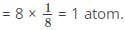Chemistry: CUET Mock Test - 1 - Question 7

Which of the following can be used to describe a crystalline solid?

Detailed Solution for Chemistry: CUET Mock Test - 1 - Question 7

Homogeneity refers to uniformity in composition, which is a characteristic property of crystalline solids. Isotropy is when the values of physical properties do not change with direction throughout the body of the solid. Crystalline solids are anisotropic because the composition of the solid changes with direction, hence the physical properties also change with direction.

Chemistry: CUET Mock Test - 1 - Question 8

Which of the following is not an example of an Ideal solution?

Detailed Solution for Chemistry: CUET Mock Test - 1 - Question 8

An ideal solution may be defined as the solution in which no volume change and no enthalpy change take place on mixing the solute and the solvent in any proportion. Ethyl alcohol + Water is a Non-Ideal solution.

Chemistry: CUET Mock Test - 1 - Question 9

What is the coordination number of a body-centered unit cell?

Detailed Solution for Chemistry: CUET Mock Test - 1 - Question 9

Coordination number of a unit cell is defined as the number of atoms/ions that surround the central atom/ion. In the case of BCC, the central particle is surrounded by 8 particles hence, 8.

Chemistry: CUET Mock Test - 1 - Question 10

The total number of atoms in one unit cell of body-centered unit cubic cell is ______ atoms.

Detailed Solution for Chemistry: CUET Mock Test - 1 - Question 10

In body-centered cubic unit cell, one atom is present at each of the corners and one atom at its body center.

•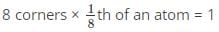• 1 body-centered atom

Thus, the total number of atoms in body-centered cubic unit cell: 1+1=2 atoms.

Chemistry: CUET Mock Test - 1 - Question 11

In a crystal, if a fault exists in the arrangement at a point, it is called as _______

Detailed Solution for Chemistry: CUET Mock Test - 1 - Question 11

When there is a fault in the arrangement of a constituent particle such as an atom, ion or molecule, and the fault exists at a point in the lattice structure, it is called as a point defect.

Chemistry: CUET Mock Test - 1 - Question 12

Which of the following is an amorphous solid?

Detailed Solution for Chemistry: CUET Mock Test - 1 - Question 12

Quartz glass does not have a perfectly ordered structure, hence it is classified as an amorphous solid. The rest are crystalline solids due to ordered structures.

Chemistry: CUET Mock Test - 1 - Question 13

Which of the following arrangements of particles does a simple cubic lattice follow?

Detailed Solution for Chemistry: CUET Mock Test - 1 - Question 13

Simple cubic lattice results from 3D close packing from 2D square-packed layers. When one 2D layer is placed on top of the other, the corresponding spheres of the second layer are exactly on top of the first one. Since both have the same, exact arrangement it is AAA type.

Chemistry: CUET Mock Test - 1 - Question 14

The total number of atoms in one unit cell of face-centered unit cubic cell is ______ atoms.

Detailed Solution for Chemistry: CUET Mock Test - 1 - Question 14

In face-centered cubic unit cell, atoms are present at each of the corners and at the centre of the face of the cube.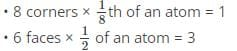Thus, the total number of atoms in face-centered cubic unit cell: 1+3=4 atoms.

Chemistry: CUET Mock Test - 1 - Question 15

In which type of point defect are the cations and anions absent in stoichiometric proportions?

Detailed Solution for Chemistry: CUET Mock Test - 1 - Question 15

A vacancy defect is when an atom, ion or molecule is absent from its position in the lattice. When this occurs for ionic solids, it is called a Schottky defect. Cations and anions are found to be absent in stoichiometric proportion so that the electrical neutrality of the crystal is maintained.

Chemistry: CUET Mock Test - 1 - Question 16

Which of the following is false regarding Non-Ideal solutions?

Detailed Solution for Chemistry: CUET Mock Test - 1 - Question 16

Non-Ideal solutions do not obey Raoult’s law. For Non-Ideal solutions, ΔVmixing ≠ 0, ΔHmixing ≠ 0. Non-ideal solutions form azeotropes or constant boiling mixtures, i.e., they have the same concentration in the vapour phase and the liquid phase.

Chemistry: CUET Mock Test - 1 - Question 17

If a crystal lattice has 6 closed-pack spheres, what the number of tetrahedral voids in the lattice?

Detailed Solution for Chemistry: CUET Mock Test - 1 - Question 17

For a crystal lattice, if there are N close-packed spheres the number of tetrahedral voids are 2N and number octahedral voids are N. For N=6, number of tetrahedral voids = 2 × 6 = 12.

Chemistry: CUET Mock Test - 1 - Question 18

Schottky defects are observed in solids with cations and anions of similar sizes. Which of the following compounds, therefore, is NOT likely to have a Schottky defect?

Detailed Solution for Chemistry: CUET Mock Test - 1 - Question 18

There is a considerable size difference between Ag cation and Cl anion. Hence a Frenkel defect is more likely to occur than a Schottky defect.

Chemistry: CUET Mock Test - 1 - Question 19

Which of the following is an example of a non-ideal solution showing positive deviation?

Detailed Solution for Chemistry: CUET Mock Test - 1 - Question 19

Chlorobenzene + Bromobenzene is an example of an ideal solution. Chloroform + Benzene and Acetone + Aniline are examples of non-ideal solutions but they show negative deviations. Acetone + Carbon disulphide is an example of a non-ideal solution showing positive deviation.

Chemistry: CUET Mock Test - 1 - Question 20

Which of the following possess anisotropic nature within their structure?

Detailed Solution for Chemistry: CUET Mock Test - 1 - Question 20

Crystalline solids possess anisotropic nature within their structure. Anisotropy is the directional dependence of a property. Meaning, a property within the crystal structure will have different values when measured in different directions. Snowflake is a crystalline solid whereas the rest are amorphous solids.

Chemistry: CUET Mock Test - 1 - Question 21

Impurity defect is a type of point defect. It can occur ________

Detailed Solution for Chemistry: CUET Mock Test - 1 - Question 21

An impurity defect occurs when a regular ion is replaced by some other type of ion, e.g. Na+ is replaced by K+. If the replacement occurs in the place of the regular cation, it is called a substitution impurity defect. If the replacement occurs in an interstitial position, it is called an interstitial impurity defect.

Chemistry: CUET Mock Test - 1 - Question 22

Which type of solid structure melts at a definite, sharp melting point?

Detailed Solution for Chemistry: CUET Mock Test - 1 - Question 22

Crystalline solids have a perfectly ordered structure which collapses immediately at a specific temperature. Amorphous solids melt over a range of temperatures, not one specific value.

Chemistry: CUET Mock Test - 1 - Question 23

Identify the dimensional relation for the unit cell illustrated below.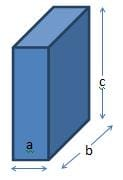Detailed Solution for Chemistry: CUET Mock Test - 1 - Question 23

The given figure represents an orthorhombic unit cell. Experimentally, it is determined that for orthorhombic unit cells a ≠ b ≠ c. All sides are unequal. It results from extension of cube along two pairs of orthogonal sides by two distinct factors.

Chemistry: CUET Mock Test - 1 - Question 24

Stainless steel is a/an ______ alloy.

Detailed Solution for Chemistry: CUET Mock Test - 1 - Question 24

Stainless steel is an interstitial alloy. Carbon atoms are introduced into interstitial spaces of iron lattice as an impurity. Further alloying sees the introduction of nickel, chromium in the interstitial spaces.

Chemistry: CUET Mock Test - 1 - Question 25

A compound is formed by atoms of elements A occupying the corners of the unit cell and an atom of element B present at the center of the unit cell. Deduce the formula of the compound.

Detailed Solution for Chemistry: CUET Mock Test - 1 - Question 25

The description is of a BCC. For BCC, each atom at the corner is shared by 8 unit cells. One atom at the center wholly belongs to the corresponding unit cell.
Therefore, total number of atoms of A present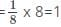Total number of atoms of B present=1
Therefore, A:B=1:1 implying the formula of the compound is AB.

Chemistry: CUET Mock Test - 1 - Question 26

Which of the following is not an example of a non-ideal solution showing negative deviation?

Detailed Solution for Chemistry: CUET Mock Test - 1 - Question 26

HNO3 + Water, HCl + Water and Acetic acid + Pyridine are non-ideal solutions showing negative deviations. Carbon tetrachloride + Toluene is an example of non-ideal solution showing positive deviation.

Chemistry: CUET Mock Test - 1 - Question 27

Atoms of element X form a BCC and atoms of element Y occupy 3/4th of the tetrahedral voids. What is the formula of the compound?

Detailed Solution for Chemistry: CUET Mock Test - 1 - Question 27

The number of tetrahedral voids form is equal to twice the number of atoms of element X. Number of atoms of Y is 3/4th the number of tetrahedral voids i.e. 3/2 times the number of atoms of X. Therefore, the ratio of numbers of atoms of X and Y are 2:3, hence X2Y3.

Chemistry: CUET Mock Test - 1 - Question 28

Which of the following is true regarding non-ideal solutions with negative deviation?

Detailed Solution for Chemistry: CUET Mock Test - 1 - Question 28

The interactions between the components of a non-ideal solution showing negative deviation are greater than the pure components. The change in volume and enthalpy after mixing is negative, i.e., ΔVmixing = -ve, ΔHmixing = -ve.

Chemistry: CUET Mock Test - 1 - Question 29

What is the total volume of the particles present in a body centered unit cell?

Detailed Solution for Chemistry: CUET Mock Test - 1 - Question 29

Since particles are assumed to be spheres and volume of one sphere is 4/3 πr3, total volume of all particles in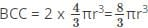since a BCC has 2 particles per cell.

Chemistry: CUET Mock Test - 1 - Question 30

Which of the following cannot form an azeotrope?

Detailed Solution for Chemistry: CUET Mock Test - 1 - Question 30

H2O + C2H5OH forms an azeotrope with a boiling point of 351.15 K. CHCl3 + C2H5 OH forms an azeotrope with a boiling point of 332.3 K. HCl + H2O forms an azeotrope with a boiling point of 383 K. Benzene + Toluene is an ideal solution and hence does not form an azeotrope.

Chemistry: CUET Mock Test - 1 - Question 31

If the aluminum unit cell exhibits face-centered behavior then how many unit cells are present in 54g of aluminum?

Detailed Solution for Chemistry: CUET Mock Test - 1 - Question 31

Atomic mass of Al = 27g/mole (contains 6.022 x 1023 Al atoms)
Since it exhibits FCC, there are 4 Al atoms/unit cell.
If 27g Al contains 6.022 x 1023 Al atoms then 54g Al contains 1.2044 x 1024atoms.
Thus, if 1 unit cell contains 4 Al atoms then number of unit cells containing 1.2044 x 1024 atoms=(1.2044 x 1024 x 1)/4 = 3.011 × 1023 unit cells.

Chemistry: CUET Mock Test - 1 - Question 32

Which of the following is true regarding azeotropes?

Detailed Solution for Chemistry: CUET Mock Test - 1 - Question 32

Azeotropes have the same concentration in the vapour phase and the liquid phase. In case of minimum boiling azeotropes, the boiling point of the azeotrope is lesser than the boiling point of either of the pure components. In case of maximum boiling azeotropes, the boiling point of the azeotrope is higher than the boiling point of either of the pure components.

Chemistry: CUET Mock Test - 1 - Question 33

What is the radius of a metal atom if it crystallizes with body-centered lattice having a unit cell edge of 333 Pico meter?

Detailed Solution for Chemistry: CUET Mock Test - 1 - Question 33

For body-centered unit cells, the relation between radius of a particle ‘r’ and edge length of unit cell ‘a’ is given as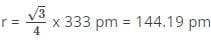is the radius of the metal atom.

Chemistry: CUET Mock Test - 1 - Question 34

If liquids A and B form an ideal solution, then what is the Gibbs free energy of mixing?

Detailed Solution for Chemistry: CUET Mock Test - 1 - Question 34

The Gibbs free energy of a system at any moment in time is defined as the enthalpy of the system minus the product of the temperature times the entropy of the system. For ideal solutions, the value of the Gibbs Free energy is always negative as mixing of ideal solutions is a spontaneous process.

Chemistry: CUET Mock Test - 1 - Question 35

How many parameters are used to characterize a unit cell?

Detailed Solution for Chemistry: CUET Mock Test - 1 - Question 35

A unit cell is characterized by six parameters i.e. the three common edge lengths a, b, c and three angles between the edges that are α, β, γ. These are referred to as inter-axial lengths and angles, respectively. The position of a unit cell can be determined by fractional coordinates along the cell edges.

Chemistry: CUET Mock Test - 1 - Question 36

5 moles of liquid X and 10 moles of liquid Y make a solution having a total vapour pressure 70 torr. The vapour pressures of pure X and pure Y are 64 torr and 76 torr respectively. Which of the following is true regarding the described solution?

Detailed Solution for Chemistry: CUET Mock Test - 1 - Question 36

Observed pressure = 76 torr
According to Raoult’s law,
pA = xA x pA0 = 5/15 x 64 = 21.33 torr
pB = xB x pB0 = 10/15 x 76 = 50.67 torr
Therefore, pressure expected by Raoult’s law = 21.33 + 50.67 = 72 torr.
Thus, observed pressure (70 torr) is less than the expected value. Hence, the solution shows negative deviation.

Chemistry: CUET Mock Test - 1 - Question 37

If a metal forms a FCC lattice with unit edge length 500 pm. Calculate the density of the metal if its atomic mass is 110.

Detailed Solution for Chemistry: CUET Mock Test - 1 - Question 37

Edge length (a) = 500 pm = 500 x 10-12 m
Atomic mass (M) = 110 g/mole = 110 x 10-3 kg/mole
Avogadro’s number (NA) = 6.022 x 1023/mole
z = 4 atoms/cell
The density, d of a metal is given as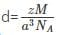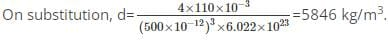Chemistry: CUET Mock Test - 1 - Question 38

What is each point (position of particle) in a crystal lattice termed as?

Detailed Solution for Chemistry: CUET Mock Test - 1 - Question 38

Each point of the particle’s position is referred to as ‘lattice point’ or ‘lattice site’. Every lattice point represents one constituent particle which may be an atom, ion or molecule.

Chemistry: CUET Mock Test - 1 - Question 39

Why is ‘raising of viscosity’ of a solution after addition of solute, not considered to be a colligative property?

Detailed Solution for Chemistry: CUET Mock Test - 1 - Question 39

A colligative property is identified by the fact that it has no dependence on the nature of the particles of solute. However, in the case of viscosity it really depends on the solute that is added to the solvent. Thus, the change in viscosity after addition of a non-volatile solute cannot be considered to be a colligative property.

Chemistry: CUET Mock Test - 1 - Question 40

At 70°C the vapor pressure of pure water is 31 kPa. Which of the following is most likely the vapor pressure of a 2.0 molal aq. glucose solution at 70°C?

Detailed Solution for Chemistry: CUET Mock Test - 1 - Question 40

Given, P0water = 31 kPa
Concentration of solution, c = 2 molal = 2 moles of glucose/kg of water
From law of relative lowering of vapor pressure, ΔP/P0 = X2, where X2 is the mole fraction of glucose in the solution.
Mass of water = 1 kg = 1000 g
Molecular weight of water = 18 g/mole
Moles of water = 1000/18 = 55.556 moles
X2 = 2/(2 + 55.556) = 0.035
ΔP = 31 kPa x 0.035 = 1.085 kPa
Final pressure = 31 kPa – 1.085 kPa = 29.915 kPa.

## CUET Science Subjects Mock Tests

60 tests
 Use Code STAYHOME200 and get INR 200 additional OFF Use Coupon Code
Information about Chemistry: CUET Mock Test - 1 Page
In this test you can find the Exam questions for Chemistry: CUET Mock Test - 1 solved & explained in the simplest way possible. Besides giving Questions and answers for Chemistry: CUET Mock Test - 1, EduRev gives you an ample number of Online tests for practice

60 tests